# TSA Exponential Average VI

LabVIEW 2014 Advanced Signal Processing Toolkit Help

Edition Date: June 2014

Part Number: 372656C-01

»View Product InfoDownload Help (Windows Only)

Owning Palette: Preprocessing VIs

Performs exponential average on a univariate or multivariate (vector) time series. This VI returns a smoothed time series. Wire data to the Xt input to determine the polymorphic instance to use or manually select the instance.

Use the pull-down menu to select an instance of this VI.

 Select an instance TSA Exponential Average (Waveform)TSA Exponential Average (Array)TSA Vector Exponential Average (Waveform)TSA Vector Exponential Average (Array)

## TSA Exponential Average (Waveform)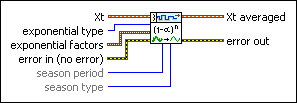Xt specifies the univariate time series.exponential type specifies the type of exponential average. Options include Single, Double, and Triple. The default is Single. Refer to the Details section for more information about each exponential type.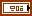exponential factors specifies the weighting factors for exponential smoothing.level specifies the weight for the level cumulant. The value must be a number between 0 and 1.trend specifies the weight for the trend cumulant. The value must be a number between 0 and 1. This option is available only when exponential type is Double or Triple.season specifies the weight for seasonal cumulant. The value must be a number between 0 and 1. This option is available only when exponential type is Triple.error in describes error conditions that occur before this node runs. This input provides standard error in functionality.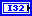season period specifies the length of the seasonal pattern in the input time series. The default is 1. This option is available only when exponential type is Triple.season type specifies the way in which this VI models the seasonality. This option is available only when exponential type is Triple. Refer to the Details section for more information about each season type.

 0 Multiplicative (default)—Indicates that the time series has a seasonality with the amplitude increasing over time. 1 Additive—Indicates that the time series has a constant amplitude change in seasonality.Xt averaged returns the averaged univariate time series.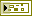error out contains error information. This output provides standard error out functionality.

## TSA Exponential Average (Array)Xt specifies the univariate time series.exponential type specifies the type of exponential average. Options include Single, Double, and Triple. The default is Single. Refer to the Details section for more information about each exponential type.exponential factors specifies the weighting factors for exponential smoothing.level specifies the weight for the level cumulant. The value must be a number between 0 and 1.trend specifies the weight for the trend cumulant. The value must be a number between 0 and 1. This option is available only when exponential type is Double or Triple.season specifies the weight for seasonal cumulant. The value must be a number between 0 and 1. This option is available only when exponential type is Triple.error in describes error conditions that occur before this node runs. This input provides standard error in functionality.season period specifies the length of the seasonal pattern in the input time series. The default is 1. This option is available only when exponential type is Triple.season type specifies the way in which this VI models the seasonality. This option is available only when exponential type is Triple. Refer to the Details section for more information about each season type.

 0 Multiplicative (default)—Indicates that the time series has a seasonality with the amplitude increasing over time. 1 Additive—Indicates that the time series has a constant amplitude change in seasonality.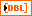Xt averaged returns the averaged univariate time series.error out contains error information. This output provides standard error out functionality.

## TSA Vector Exponential Average (Waveform)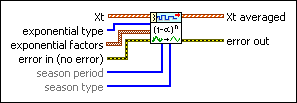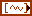Xt specifies the multivariate (vector) time series.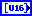exponential type specifies the type of exponential average. Each element of the array is one selection for one channel. Refer to the Details section for more information about each exponential type.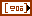exponential factors specifies the weighting factors for exponential smoothing. Each element of the array represents one selection for one channel.level specifies the weight for the level cumulant. The value must be a number between 0 and 1.trend specifies the weight for the trend cumulant. The value must be a number between 0 and 1. This option is available only when exponential type is Double or Triple.season specifies the weight for seasonal cumulant. The value must be a number between 0 and 1. This option is available only when exponential type is Triple.error in describes error conditions that occur before this node runs. This input provides standard error in functionality.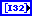season period specifies the length of the seasonal pattern in the input time series. Each element of the array represents one selection for one channel. This option is available only when exponential type is Triple.season type specifies the way in which this VI models the seasonality. Each element of the array represents one selection for one channel. This option is available only when exponential type is Triple. Refer to the Details section for more information about each season type.Xt averaged returns the averaged multivariate (vector) time series.error out contains error information. This output provides standard error out functionality.

## TSA Vector Exponential Average (Array)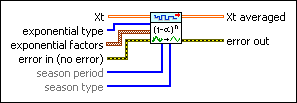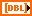Xt specifies the multivariate (vector) time series. Each column of the 2D array represents a vector at certain time.exponential type specifies the type of exponential average. Each element of the array is one selection for one channel. Refer to the Details section for more information about each exponential type.exponential factors specifies the weighting factors for exponential smoothing. Each element of the array represents one selection for one channel.level specifies the weight for the level cumulant. The value must be a number between 0 and 1.trend specifies the weight for the trend cumulant. The value must be a number between 0 and 1. This option is available only when exponential type is Double or Triple.season specifies the weight for seasonal cumulant. The value must be a number between 0 and 1. This option is available only when exponential type is Triple.error in describes error conditions that occur before this node runs. This input provides standard error in functionality.season period specifies the length of the seasonal pattern in the input time series. Each element of the array represents one selection for one channel. This option is available only when exponential type is Triple.season type specifies the way in which this VI models the seasonality. Each element of the array represents one selection for one channel. This option is available only when exponential type is Triple. Refer to the Details section for more information about each season type.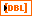Xt averaged returns the averaged multivariate (vector) time series. Each column of the 2D array represents a vector at certain time.error out contains error information. This output provides standard error out functionality.

## TSA Exponential Average Details

This VI uses the exponential weighting scheme to produce a smoothed time series. This VI computes the averaged values by assigning exponentially decreasing weights to the old values in the original time series according to the following equation:

Xa(i) = aXt(i–1) + a(1–a)Xt(i–2) + a(1–a)2Xt(i–3) + …

where a is the level weight for level cumulant Xa, Xa is the averaged time series, and Xt is the original time series.

### Single

This average scheme begins by initializing Xa(0) as Xt(0), For any time period i, this VI computes the averaged value Xa according to the following equation:

Xa(i) = aXt(i) + (1–a)Xa(i–1), 0<a<1, n≥2

### Double

This average scheme begins by initializing Xa(0) as Xt(0), For any time period i, this VI computes the averaged value Xa according to the following equation:

Xa(i) = aXt(i) + (1–a)[Xa(i–1) + B(i–1)], 0<a<1

B(i) = b[Xa(i)–Xa(i–1)]+(1–b)B(i–1), 0<b<1

where b is the trend weight for trend cumulant B. B(0) is initialized as Xt(1)–Xt(0).

### Triple

This average scheme begins by initializing Xa(0) as Xt(0) and computes different averaged values depending on the seasonality type: Multiplicative or Additive.

• Multiplicative seasonality

For any time period i, this VI computes the averaged value Xa according to the following equation: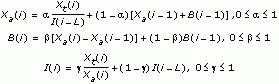where b is the trend weight for trend cumulant B, g is the season weight for season cumulant vector I, and L is season period.

For any time period i, this VI computes the averaged value Xa according to the following equation: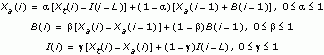B(0) is initialized as follows:Ij, j=–L, …, –1 is initialized as follows: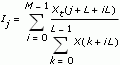M is approximately equal to the length of the time series divided by L, and I0=I-L

### Reference:

NIST/SEMATECH e-Handbook of Statistical Methods, http://www.itl.nist.gov/div898/handbook, 2005.

## Example

Refer to the Exponential Smoothing VI in the labview\examples\Time Series Analysis\TSAGettingStarted directory for an example of using the TSA Exponential Average VI.